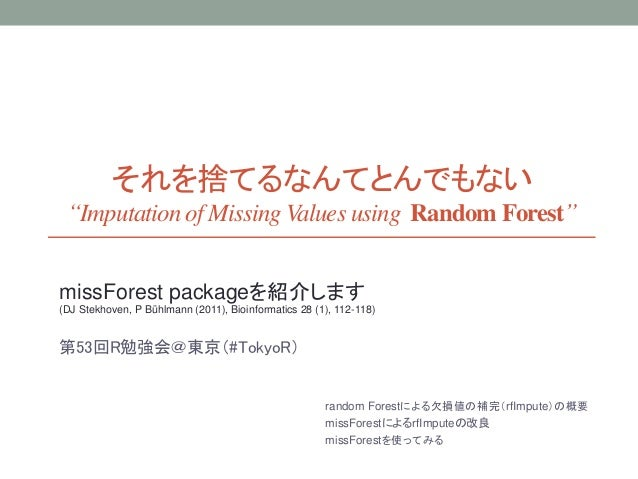Successfully reported this slideshow.Upcoming SlideShare
×

# Imputation of Missing Values using Random Forest

6,371 views

Published on

missForest packageの紹介
“MissForest - nonparametric missing value imputation for mixed-type data (DJ Stekhoven, P Bühlmann (2011), Bioinformatics 28 (1), 112-118)

Published in: Data & Analytics
• Full Name
Comment goes here.

Are you sure you want to Yes No• Dating direct: ❤❤❤ http://bit.ly/2Qu6Caa ❤❤❤

Are you sure you want to  Yes  No

Are you sure you want to  Yes  No

Are you sure you want to  Yes  No

Are you sure you want to  Yes  No

### Imputation of Missing Values using Random Forest

1. 1. それを捨てるなんてとんでもない “Imputation of Missing Values using Random Forest” missForest packageを紹介します (DJ Stekhoven, P Bühlmann (2011), Bioinformatics 28 (1), 112-118) 第53回R勉強会＠東京（#TokyoR） random Forestによる欠損値の補完（rfImpute）の概要 missForestによるrfImputeの改良 missForestを使ってみる
2. 2. 欠測のある観測データ、どうしてますか？ https://www.iwanami.co.jp/cgi-bin/isearch?isbn=ISBN978-4-00-029847-6 目次 1. はじめに：欠測のDo's and Don'ts とガイドライン 2. 欠測データに対する最尤法 3. EMアルゴリズム 4. 単一代入と多重代入 5. 回帰分析モデルにおける欠測データ解析 6. 脱落を伴う経時測定データの解析 7. 欠測データメカニズムの検討
3. 3. ランダムフォレスト 学習データのランダムサブセットで構築した様々な決定木の集合（＝森）の 予測結果 を統合する  分類 → 多数決  回帰 → 平均  特定の説明変数への依存が少ないため、クエリデータの説明変数が 欠損していても良い出力を与える ALL DATA Random subset Random subset Random subset …
4. 4. データ ①，② データ ③ 弱学習器＝(決定木) 欠損値を初期値で埋める  連続変数 → 中央値  カテゴリカル変数 → 最頻値 それぞれの木でデータ同士の近似度計算  目的変数が同じLeafに落ちたら＋１  そうでなければ＋０ 森全体でデータ間の近似度を集計・正規化  近似度の和÷木の数 欠損していない他のデータから補完 連続変数 → 近似度による重み付け平均値 カテゴリカル変数 → 近似度による重みづけ最頻値データ①と②の近似度は＋１ データ①と③の近似度は＋０ randomForestで学習  ノンパラメトリックな補完  連続・カテゴリカルな変数の混在を許す random Forestによる欠損値の補完 {randomForest} パッケージには、rfImpute 関数が用意されている
5. 5. 目的変数が同じLeafに落ちるかどうかで重みを計算する ↓ 目的変数も欠損しているデータには 適用できない 既知のデータセットに含まれる欠損値の推定には役立つが、 予測をしたいデータの前処理に用いたいときに困る random Forestによる欠損値の補完 {randomForest} パッケージには、rfImpute 関数が用意されているが…
6. 6. random Forestによる欠損値の補完 missForestの改良点 補完したい変数を目的変数に設定する 変数ごとに予測値で補完 目的変数の欠損の有無でデータ分割  補完したい変数が非欠損 → 教師データ  補完したい変数が欠損値 → テストデータ テストデータ＝欠損値の予測 教師データでrandomForestの学習 補完開始
7. 7. missForestを使ってみる データの準備 データの 準備 とりあえず 動かす （検証） エラー計測と チューニング #install.packages(missForest, dependencies = TRUE) require(dplyr) require(missForest) data(diamonds, package = "ggplot2") dia.sample <- sample_n(diamonds, size=2000) dia.sample <- as.data.frame(dia.sample) summary(dia.sample) dia.sample %>% head par(mfrow=c(3,4)) for(i in c(1,5:10)){ hist(unlist(dia.sample[,i]) , main=colnames(dia.sample[,i]), xlab="") } plot.new() for(i in 2:4){ dia.sample[,i] %>% table %>% barplot } par(mfrow=c(1,1))  連続変数 ×７  カテゴリ変数 ×３ ggplot2のdiamondデータを間引いて使う
8. 8. missForestを使ってみる データの準備 データの 準備 とりあえず 動かす （検証） エラー計測と チューニング # create sample data with NA --------------------------------------------- dia.mis <- prodNA(dia.sample, noNA=0.05) summary(dia.mis) ５％欠損データを人工的につくる
9. 9. missForestを使ってみる とりあえず動かす データの 準備 とりあえず 動かす （検証） エラー計測と チューニング dia.imp <- missForest(dia.mis, verbose=TRUE) dia.imp %>% str(max.level=1) dia.imp\$ximp 返ってくる結果の構造に注意！ 補完後のデータは \$ximp ※ よく間違える Iterationの打ち切り条件  defference(s) が十分に小さくなったら収束
10. 10. missForestを使ってみる エラー計測： OOB error※を算出し、補完精度を推定する データの 準備 とりあえず 動かす （検証） エラー計測と チューニング dia.imp\$OOBerror dia.imp <- missForest(dia.mis, verbose=TRUE, variablewise=TRUE) ※ out-of-bag (OOB) imputation error estimate 連続変数全体の評価＝NRMSE （normalized root mean squared error） カテゴリ変数全体の評価＝PFC （proportion of falsely classified ） TRUEとセットすると変数の数だけ OOB errorを返す（並びはカラム順）
11. 11. missForestを使ってみる チューニング： OOB errorを見ながら変えてみる。基本はRFと同じ。 データの 準備 とりあえず 動かす （検証） エラー計測と チューニング dia.imp <- missForest(dia.mis, verbose=TRUE, maxiter=4) 補完の繰り返し回数 ※ 収束するまで繰り返しがデフォルト推奨 dia.imp <- missForest(dia.mis, verbose=TRUE, mtree=4000) 決定木の数 ※ 多いほうがよさそうだが、 増やした分だけ計算量は線形に増加する dia.imp <- missForest(dia.mis, verbose=TRUE, mtry=4) 決定木あたりに使う変数の数 ※ デフォルトは（変数の数）1/2だが、 推奨値の提示は難しい（データ次第）とのこと
12. 12. missForestを使ってみる 変数ごとに補完プロセスを並列化 データの 準備 エラー計測と チューニング 補完プロセスの 並列化 require(doParallel) cl <- makeCluster(NCOL(dia.mis)) registerDoParallel(cl) dia.imp <- missForest( xmis = dia.mis, variablewise = TRUE, ntree = 1200, parallelize = "variables", verbose = TRUE) stopCluster(cl) （検証）
13. 13. missForestを使ってみる randomForestの学習を並列化 データの 準備 エラー計測と チューニング 補完プロセスの 並列化 require(doParallel) cl <- makeCluster(NCOL(dia.mis)) registerDoParallel(cl) dia.imp <- missForest( xmis = dia.mis, variablewise = TRUE, ntree = 1200, parallelize = "forests", verbose = TRUE) stopCluster(cl) ※ 注意 ソースコードを見ると指定できるコア数に制約あり makeClusterで指定するコア数≦カラムの数 （variablewiseな並列化を推奨？） （検証）
14. 14. missForestを使ってみる 検証： 欠損のない完全データがあるときには補完精度の評価ができる データの 準備 補完プロセス （検証） エラー計測と チューニング mixError(ximp = dia.imp\$ximp, xmis = dia.mis, xtrue= dia.sample) dia.imp <- missForest(dia.mis, verbose=TRUE, xtrue=dia.sample) ximp ： 補完後のデータ xmis ： 人工的に作った欠損データ xtrue： 欠損のない完全データ 補完中の精度評価も可能
15. 15. missForestを使ってみる 検証： mixError()は OOB Errorを基準に、別の補完法との比較もできる require(mi) dia.mdf <- missing_data.frame(dia.mis) summary(dia.mdf) n.chains <- 20 options(mc.cores=n.chains) dia.MI <- mi(dia.mdf, n.chains = n.chains) str(dia.MI,max.level = 2) dia.dfs <- complete(dia.MI) str(dia.dfs) dia.imp_itr <- foreach(i=1:n.chains) %do% { this <- dia.dfs[[i]]%>%select(-contains("missing")) } dia.err_mi <- foreach(i=1:n.chains, .combine=rbind) %do% { mixError(ximp = dia.imp_itr[[i]], xmis = dia.mis, xtrue= dia.sample) } dia.err_mi colMeans(dia.err_mi) データの 準備 補完プロセス （検証） エラー計測と チューニング
16. 16. 参考文献 • randomForest {randomForest} • Breiman, L. (2001). Random forests. Machine learning, 45(1), 5–32. • cForest {party} • "Party on! A New, Conditional Variable Importance Measure for Random Forests Available in the party Package", Strobl et al. 2009. • http://epub.ub.uni-muenchen.de/9387/1/techreport.pdf • rfImputeによる欠損値の補完 • “Random Forestを用いた欠測データの補完とその応用” • http://www.rd.dnc.ac.jp/~tunenori/doc/jjasRf2010slide.pdf • missForest • Package missForest • https://cran.r-project.org/web/packages/missForest/ • Vignette: “Using the missForest Package” • https://stat.ethz.ch/education/semesters/ss2013/ams/paper/missForest_1.2.pdf • “MissForest - nonparametric missing value imputation for mixed-type data” • http://bioinformatics.oxfordjournals.org/content/early/2011/10/28/bioinformatics.btr597.full.pdf